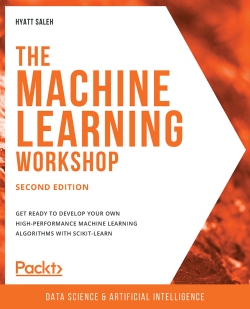### The Machine Learning Workshop - Second Edition

By Hyatt Saleh
• Constantly updated with 100+ new titles each month
• Breadth and depth in over 1,000+ technologies

Machine learning algorithms are an integral part of almost all modern applications. To make the learning process faster and more accurate, you need a tool flexible and powerful enough to help you build machine learning algorithms quickly and easily. With The Machine Learning Workshop, you'll master the scikit-learn library and become proficient in developing clever machine learning algorithms.

The Machine Learning Workshop begins by demonstrating how unsupervised and supervised learning algorithms work by analyzing a real-world dataset of wholesale customers. Once you've got to grips with the basics, you’ll develop an artificial neural network using scikit-learn and then improve its performance by fine-tuning hyperparameters. Towards the end of the workshop, you'll study the dataset of a bank's marketing activities and build machine learning models that can list clients who are likely to subscribe to a term deposit. You'll also learn how to compare these models and select the optimal one.

By the end of The Machine Learning Workshop, you'll not only have learned the difference between supervised and unsupervised models and their applications in the real world, but you'll also have developed the skills required to get started with programming your very own machine learning algorithms.

Publication date:
July 2020
Publisher
Packt
Pages
286
ISBN
9781839219061

# 1. Introduction to Scikit-Learn

Overview

This chapter introduces the two main topics of this book: machine learning and scikit-learn. By reading this book, you will learn about the concept and application of machine learning. You will also learn about the importance of data in machine learning, as well as the key aspects of data preprocessing to solve a variety of data problems. This chapter will also cover the basic syntax of scikit-learn. By the end of this chapter, you will have a firm understanding of scikit-learn's syntax so that you can solve simple data problems, which will be the starting point for developing machine learning solutions.

# Introduction

Machine learning (ML), without a doubt, is one of the most relevant technologies nowadays as it aims to convert information (data) into knowledge that can be used to make informed decisions. In this chapter, you will learn about the different applications of ML in today's world, as well as the role that data plays. This will be the starting point for introducing different data problems throughout this book that you will be able to solve using scikit-learn.

Scikit-learn is a well-documented and easy-to-use library that facilitates the application of ML algorithms by using simple methods, which ultimately enables beginners to model data without the need for deep knowledge of the math behind the algorithms. Additionally, thanks to the ease of use of this library, it allows the user to implement different approximations (that is, create different models) for a data problem. Moreover, by removing the task of coding the algorithm, scikit-learn allows teams to focus their attention on analyzing the results of the model to arrive at crucial conclusions.

Spotify, a world-leading company in the field of music streaming, uses scikit-learn because it allows them to implement multiple models for a data problem, which are then easily connected to their existing development. This process improves the process of arriving at a useful model, while allowing the company to plug them into their current app with little effort.

On the other hand, booking.com uses scikit-learn due to the wide variety of algorithms that the library offers, which allows them to fulfill the different data analysis tasks that the company relies on, such as building recommendation engines, detecting fraudulent activities, and managing the customer service team.

Considering the preceding points, this chapter also explains scikit-learn and its main uses and advantages, and then moves on to provide a brief explanation of the scikit-learn Application Programming Interface (API) syntax and features. Additionally, the process of representing, visualizing, and normalizing data will be shown. The aforementioned information will help us to understand the different steps that need to be taken to develop a ML model.

In the following chapters in this book, you will explore the main ML algorithms that can be used to solve real-life data problems. You will also learn about different techniques that you can use to measure the performance of your algorithms and how to improve them accordingly. Finally, you will explore how to make use of a trained model by saving it, loading it, and creating APIs.

# Introduction to Machine Learning

Machine learning (ML) is a subset of Artificial Intelligence (AI) that consists of a wide variety of algorithms capable of learning from the data that is being fed to them, without being specifically programmed for a task. This ability to learn from data allows the algorithms to create models that are capable of solving complex data problems by finding patterns in historical data and improving them as new data is fed to the models.

These different ML algorithms use different approximations to solve a task (such as probability functions), but the key element is that they are able to consider a countless number of variables for a particular data problem, making the final model better at solving the task than humans are. The models that are created using ML algorithms are created to find patterns in the input data so that those patterns can be used to make informed predictions in the future.

## Applications of ML

Some of the popular tasks that can be solved using ML algorithms are price/demand predictions, product/service recommendation, and data filtering, among others. The following is a list of real-life examples of such tasks:

• On-demand price prediction: Companies whose services vary in price according to demand can use ML algorithms to predict future demand and determine whether they will have the capability to meet it. For instance, in the transportation industry, if future demand is low (low season), the price for flights will drop. On the other hand, is demand is high (high season), flights are likely to increase in price.
• Recommendations in entertainment: Using the music that you currently use, as well as that of the people similar to you, ML algorithms can construct models capable of suggesting new records that you may like. That is also the case of video streaming applications, as well as online bookstores.
• Email filtering: ML has been used for a while now in the process of filtering incoming emails in order to separate spam from your desired emails. Lately, it also has the capability to sort unwanted emails into more categories, such as social and promotions.

## Choosing the Right ML Algorithm

When it comes to developing ML solutions, it is important to highlight that, more often than not, there is no one solution for a data problem, much like there is no algorithm that fits all data problems. According to this and considering that there is a large quantity of algorithms in the field of ML, choosing the right one for a certain data problem is often the turning point that separates outstanding models from mediocre ones.

The following steps can help narrow down the algorithms to just a few:

1. Understand your data: Considering that data is the key to being able to develop any ML solutions, the first step should always be to understand it in order to be able to filter out any algorithm that is unable to process such data.

For instance, considering the quantity of features and observations in your dataset, it is possible to determine whether an algorithm capable of producing outstanding results with a small dataset is required. The number of instances/features to consider a dataset small depends on the data problem, the quantity of the outputs, and so on. Moreover, by understanding the types of fields in your dataset, you will also be able to determine whether you need an algorithm capable of working with categorical data.

2. Categorize the data problem: As per the following diagram, in this step, you should analyze your input data to determine if it contains a target feature (a feature whose values you want to be modeled and predicted) or not. Datasets with a target feature are also known as labeled data and are solved using supervised learning (`A`) algorithms. On the other hand, datasets without a target feature are known as unlabeled data and are solved using unsupervised learning algorithms (`B`).

Moreover, the output data (the form of output that you expect from the model) also plays a key role in determining the algorithms to be used. If the output from the model needs to be a continuous number, the task to be solved is a regression problem (`C`). On the other hand, if the output is a discrete value (a set of categories, for instance), the task at hand is a classification problem (`D`). Finally, if the output is a subgroup of observations, the process to be performed is a clustering task (`E`):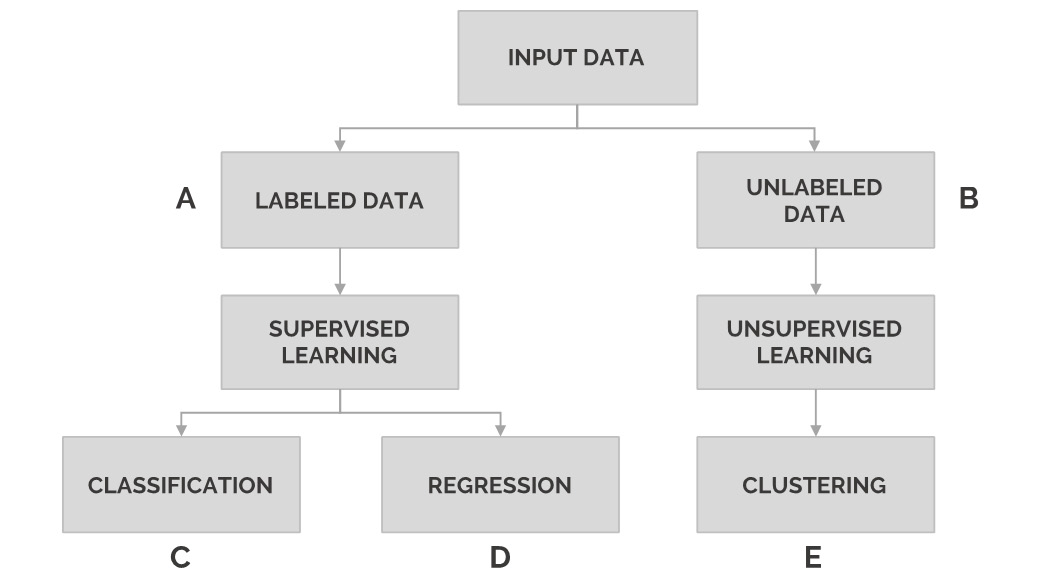Figure 1.1: Demonstrating the division of tasks

This division of tasks will be explored in more detail in the Supervised and Unsupervised Learning section of this chapter.

3. Choose a set of algorithms: Once the preceding steps have been performed, it is possible to filter out the algorithms that perform well over the input data and that are able to arrive at the desired outcome. Depending on your resources and time limitations, you should choose from this list of apt algorithms the ones that you want to test out over your data problem, considering that it is always a good practice to try more than one algorithm.

These steps will be explained in more detail in the next chapter using a real-life data problem as an example.

# Scikit-Learn

Created in 2007 by David Cournapeau as part of a Google Summer of Code project, scikit-learn is an open source Python library made to facilitate the process of building models based on built-in ML and statistical algorithms, without the need for hardcoding. The main reasons for its popular use are its complete documentation, its easy-to-use API, and the many collaborators who work every day to improve the library.

Note

You can find the documentation for scikit-learn at http://scikit-learn.org.

Scikit-learn is mainly used to model data, and not as much to manipulate or summarize data. It offers its users an easy-to-use, uniform API to apply different models with little learning effort, and no real knowledge of the math behind it is required.

Note

Some of the math topics that you need to know about to understand the models are linear algebra, probability theory, and multivariate calculus. For more information on these models, visit https://towardsdatascience.com/the-mathematics-of-machine-learning-894f046c568.

The models that are available in the scikit-learn library fall into two categories, that is, supervised and unsupervised, both of which will be explained in depth later in this chapter. This form of category classification will help to determine which model to use for a particular dataset to get the most information out of it.

Besides its main use for predicting future behavior in supervised learning problems and clustering data in unsupervised learning problems, scikit-learn is also used for the following reasons:

• To carry out cross-validation and performance metrics analysis to understand the results that have been obtained from the model, and thereby improve its performance
• To obtain sample datasets to test algorithms on them
• To perform feature extraction to extract features from images or text data

Although scikit-learn is considered the preferred Python library for beginners in the world of ML, there are several large companies around the world that use it because it allows them to improve their products or services by applying the models to already existing developments. It also permits them to quickly implement tests on new ideas.

Some of the leading companies that are using scikit-learn are as follows:

• Spotify: One of the most popular music streaming applications, Spotify makes use of scikit-learn mainly due to the wide variety of algorithms that the framework offers, as well as how easy it is to implement the new models into their current developments. Scikit-learn has been used as part of its music recommendation model.
• Booking.com: From developing recommendation systems to preventing fraudulent activities, among many other solutions, this travel metasearch engine has been able to use scikit-learn to explore a large number of algorithms that allow the creation of state-of-the-art models.
• Evernote: This note-taking and management app uses scikit-learn to tackle several of the steps required to train a classification model, from data exploration to model evaluation.
• Change.org: Thanks to the framework's ease of use and variety of algorithms, this non-profit organization has been able to create email marketing campaigns that reach millions of readers around the world.

Note

You can visit http://scikit-learn.org/stable/testimonials/testimonials.html to discover other companies that are using scikit-learn and see what they are using it for.

In conclusion, scikit-learn is an open source Python library that uses an API to apply most ML tasks (both supervised and unsupervised) to data problems. Its main use is for modeling data so that predictions can be made about unseen observations; nevertheless, it should not be limited to that as the library also allows users to predict outcomes based on the model being trained, as well as to analyze the performance of the model, among other features.

The following is a list of the main advantages of using scikit-learn for ML purposes:

• Ease of use: Scikit-learn is characterized by a clean API, with a small learning curve in comparison to other libraries, such as TensorFlow or Keras. The API is popular for its uniformity and straightforward approach. Users of scikit-learn do not necessarily need to understand the math behind the models.
• Uniformity: Its uniform API makes it very easy to switch from model to model as the basic syntax that's required for one model is the same for others.
• Documentation/tutorials: The library is completely backed up by documentation, which is effortlessly accessible and easy to understand. Additionally, it also offers step-by-step tutorials that cover all of the topics required to develop any ML project.
• Reliability and collaborations: As an open source library, scikit-learn benefits from the input of multiple collaborators who work each day to improve its performance. This participation from many experts from different contexts helps to develop not only a more complete library but also a more reliable one.
• Coverage: As you scan the list of components that the library has, you will discover that it covers most ML tasks, ranging from supervised models such as performing a regression task to unsupervised models such as the ones used to cluster data into subgroups. Moreover, due to its many collaborators, new models tend to be added in relatively short amounts of time.

The following is a list of the main disadvantages of using scikit-learn for ML purposes:

• Inflexibility: Due to its ease of use, the library tends to be inflexible. This means that users do not have much liberty in parameter tuning or model architecture, such as with the Gradient Boost algorithm and neural networks. This becomes an issue as beginners move to more complex projects.
• Not good for deep learning: The performance of the library falls short when tackling complex ML projects. This is especially true for deep learning, as scikit-learn does not support deep neural networks with the necessary architecture or power.

Note

Deep learning is a part of ML and is based on the concept of artificial neural networks. It uses a sequence of layers to extract valuable information (features) from the input data. In subsequent sections of this book, you will learn about neural networks, which is the starting point of being able to develop deep learning solutions.

In general terms, scikit-learn is an excellent beginner's library as it requires little effort to learn its use and has many complementary materials thought to facilitate its application. Due to the contributions of several collaborators, the library stays up to date and is applicable to most current data problems.

On the other hand, it is a simple library that's not fit for more complex data problems such as deep learning. Likewise, it is not recommended for users who wish to take their abilities to a higher level by playing with the different parameters that are available in each model.

## Other Frameworks

Other popular ML frameworks are as follows:

• TensorFlow: Google's open source framework for ML, which to this day is still the most popular among data scientists. It is typically integrated with Python and is very good for developing deep learning solutions. Due to its popularity, the information that's available on the internet about the framework makes it very easy to develop different solutions, not to mention that it is backed by Google.
• PyTorch: This was primarily developed by Facebook's AI Research lab as an open source deep learning framework. Although it is a fairly new framework (released in 2017), it has grown in popularity due to its ease of use and Pythonic nature. It allows easy code debugging thanks to the use of dynamic graph computations.
• Keras: This is an open source deep learning framework that's typically good for those who are just starting out. Due to its simplicity, it is less flexible but ideal for prototyping simple concepts. Similar to scikit-learn, it has its own easy-to-use API.

# Data Representation

The main objective of ML is to build models by interpreting data. To do so, it is highly important to feed the data in a way that is readable by the computer. To feed data into a scikit-learn model, it must be represented as a table or matrix of the required dimensions, which we will discuss in the following section.

## Tables of Data

Most tables that are fed into ML problems are two-dimensional, meaning that they contain rows and columns. Conventionally, each row represents an observation (an instance), whereas each column represents a characteristic (feature) of each observation.

The following table is a fragment of a sample dataset of scikit-learn. The purpose of the dataset is to differentiate from among three types of iris plants based on their characteristics. Hence, in the following table, each row embodies a plant and each column denotes the value of that feature for every plant: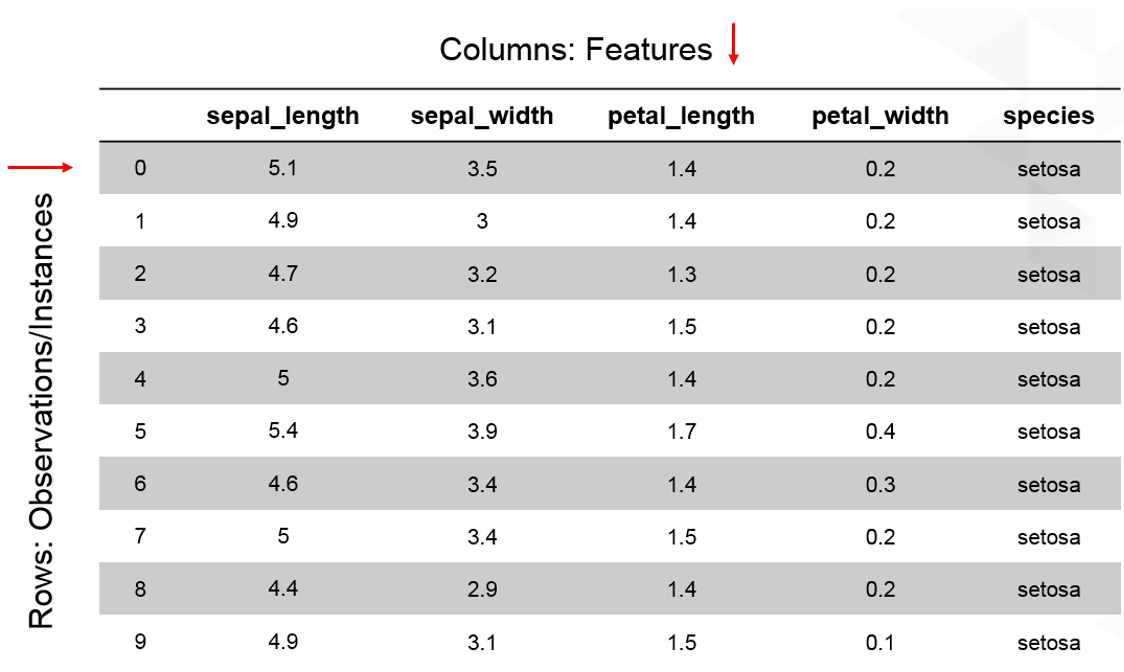Figure 1.2: A table showing the first 10 instances of the iris dataset

From the preceding explanation, by reviewing the first row of the preceding table, it is possible to determine that the observation corresponds to that of a plant with a sepal length of `5.1`, a sepal width of `3.5`, a petal length of `1.4`, and a petal width of `0.2`. The plant belongs to the `setosa` species.

Note

When feeding images to a model, the tables become three-dimensional, where the rows and columns represent the dimensions of the image in pixels, while the depth represents its color scheme. If you are interested, feel free to find out more about convolutional neural networks.

Data in tables are also known as structured data. Unstructured data, on the other hand, refers to everything else that cannot be stored in a table-like database (that is, in rows and columns). This includes images, audio, videos, and text (such as emails or reviews). To be able to feed unstructured data into an ML algorithm, the first step should be to transform it into a format that the algorithm can understand (tables of data). For instance, images are converted into matrices of pixels, and text is encoded into numeric values.

## Features and Target Matrices

For many data problems, one of the features of your dataset will be used as a label. This means that out of all the other features, this one is the target that the model should generalize the data to. For example, in the preceding table, we might choose the species as the target feature, so we would like the model to find patterns based on the other features to determine whether a plant belongs to the `setosa` species. Therefore, it is important to learn how to separate the target matrix from the features matrix.

Features Matrix: The features matrix comprises data from each instance for all features, except the target. It can be either created using a NumPy array or a Pandas DataFrame, and its dimensions are `[n_i, n_f]`, where `n_i` denotes the number of instances (such as the universe of persons in the dataset) and `n_f` denotes the number of features (such as the demographics of each person). Generally, the features matrix is stored in a variable named `X`.

Note

Pandas is an open source library built for Python. It was created to tackle different tasks related to data manipulation and analysis. Likewise, NumPy an open source Python library and is used to manipulate large multi-dimensional arrays. It was also created with a large set of mathematical functions to operate over such arrays.

Target Matrix: Different to the features matrix, the target matrix is usually one-dimensional since it only carries one feature for all instances, meaning that its length is `n_i` (the number of instances). Nevertheless, there are some occasions where multiple targets are required, so the dimensions of the matrix become `[n_i, n_t]`, where `n_t` is the number of targets to consider.

Similar to the features matrix, the target matrix is usually created as a NumPy array or a Pandas series. The values of the target array may be discrete or continuous. Generally, the target matrix is stored in a variable named `Y`.

## Exercise 1.01: Loading a Sample Dataset and Creating the Features and Target Matrices

Note

All of the exercises and activities in this book will be primarily developed in Jupyter Notebooks. It is recommended to keep a separate Notebook for different assignments, unless advised otherwise. Also, to load a sample dataset, the `seaborn` library will be used, as it displays the data as a table. Other ways to load data will be explained in later sections.

In this exercise, we will be loading the `tips` dataset from the `seaborn` library and creating features and target matrices using it. Follow these steps to complete this exercise:

Note

For the exercises and activities within this chapter, ensure that you have Python 3.7, Seaborn 0.9, Jupyter 6.0, Matplotlib 3.1, NumPy 1.18, and Pandas 0.25 installed on your system.

1. Open a Jupyter Notebook to complete this exercise. In the Command Prompt or Terminal, navigate to the desired path and use the following command:
`jupyter notebook`
2. Load the `tips` dataset using the `seaborn` library. To do so, you need to import the `seaborn` library and then use the `load_dataset()` function, as shown in the following code:
```import seaborn as sns

As we can see from the preceding code, after importing the library, a nickname is given to facilitate its use with the script.

The `load_dataset()` function loads datasets from an online repository. The data from the dataset is stored in a variable named `tips`.

3. Create a variable, `X`, to store the features. Use the `drop()` function to include all of the features but the target, which in this case is named `tip`. Then, print out the top 10 instances of the variable:
```X = tips.drop('tip', axis=1)

Note

The `axis` parameter in the preceding snippet denotes whether you want to drop the label from rows (`axis = 0`) or columns (`axis = 1`).

The printed output should look as follows: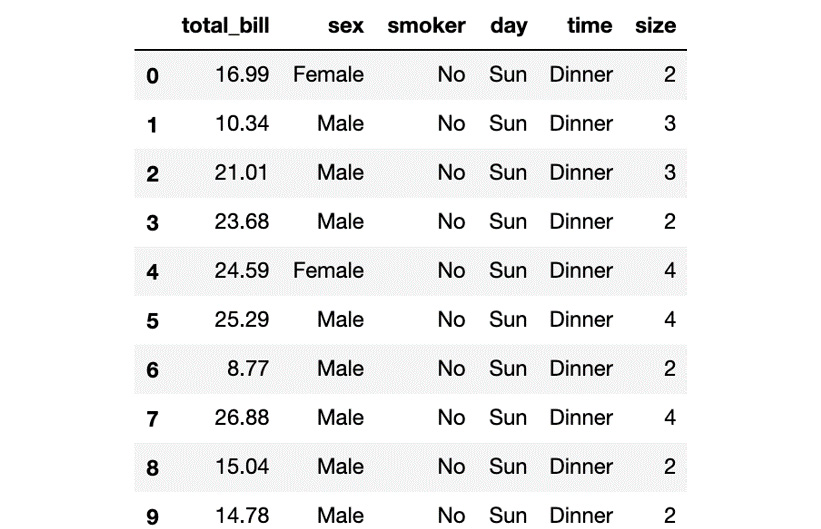Figure 1.3: A table showing the first 10 instances of the features matrix

4. Print the shape of your new variable using the `X.shape` command:
`X.shape`

The output is as follows:

`(244, 6)`

The first value indicates the number of instances in the dataset (`244`), while the second value represents the number of features (`6`).

5. Create a variable, `Y`, that will store the target values. There is no need to use a function for this. Use indexing to grab only the desired column. Indexing allows you to access a section of a larger element. In this case, we want to grab the column named `tip`. Then, we need to print out the top 10 values of the variable:
```Y = tips['tip']

The printed output should look as follows: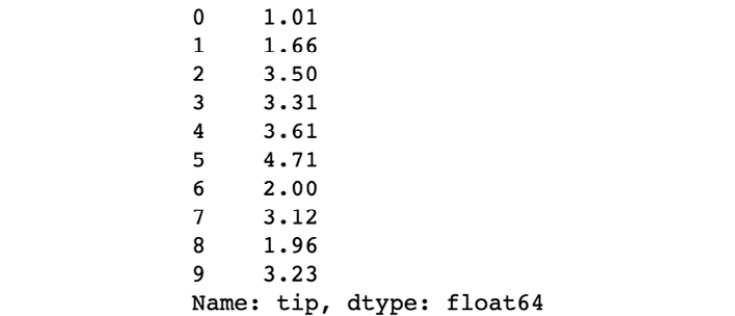Figure 1.4: A screenshot showing the first 10 instances of the target matrix

6. Print the shape of your new variable using the `Y.shape` command:
`Y.shape`

The output is as follows:

`(244,) `

The shape should be one-dimensional with a length equal to the number of instances (`244`).

Note

To access the source code for this specific section, please refer to https://packt.live/2Y5dgZH.

You can also run this example online at https://packt.live/3d0Hsco. You must execute the entire Notebook in order to get the desired result.

With that, you have successfully created the features and target matrices of a dataset.

Generally, the preferred way to represent data is by using two-dimensional tables, where the rows represent the number of observations, also known as instances, and the columns represent the characteristics of those instances, commonly known as features.

For data problems that require target labels, the data table needs to be partitioned into a features matrix and a target matrix. The features matrix will contain the values of all features but the target, for each instance, making it a two-dimensional matrix. On the other hand, the target matrix will only contain the value of the target feature for all entries, making it a one-dimensional matrix.

## Activity 1.01: Selecting a Target Feature and Creating a Target Matrix

You want to analyze the Titanic dataset to see the survival rate of the passengers on different decks and see if you can prove a hypothesis stating that passengers on the lower decks were less likely to survive. In this activity, we will attempt to load a dataset and create the features and target matrices by choosing the appropriate target feature for the objective at hand.

Note

To choose the target feature, remember that the target should be the outcome that we want to interpret the data for. For instance, if we want to know what features play a role in determining a plant's species, the species should be the target value.

Follow these steps to complete this activity:

1. Load the `titanic` dataset using the `seaborn` library. The first couple of rows should look like this: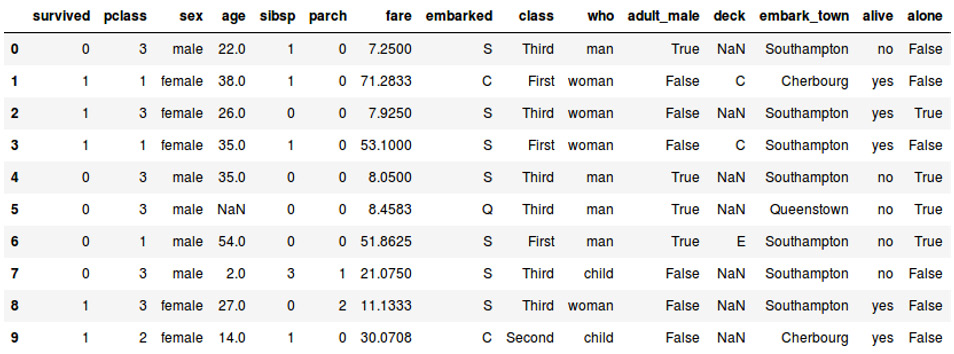Figure 1.5: A table showing the first 10 instances of the Titanic dataset

2. Select your preferred target feature for the goal of this activity.
3. Create both the features matrix and the target matrix. Make sure that you store the data from the features matrix in a variable, `X`, and the data from the target matrix in another variable, `Y`.
4. Print out the shape of each of the matrices, which should match the following values:
```Features matrix: (891, 14)
Target matrix: (891,)```

Note

The solution for this activity can be found on page 210.

# Data Preprocessing

Data preprocessing is a very critical step for developing ML solutions as it helps make sure that the model is not trained on biased data. It has the capability to improve a model's performance, and it is often the reason why the same algorithm for the same data problem works better for a programmer that has done an outstanding job preprocessing the dataset.

For the computer to be able to understand the data proficiently, it is necessary to not only feed the data in a standardized way but also make sure that the data does not contain outliers or noisy data, or even missing entries. This is important because failing to do so might result in the algorithm making assumptions that are not true to the data. This will cause the model to train at a slower pace and to be less accurate due to misleading interpretations of data.

Moreover, data preprocessing does not end there. Models do not work the same way, and each one makes different assumptions. This means that we need to preprocess the data in terms of the model that is going to be used. For example, some models accept only numerical data, whereas others work with nominal and numerical data.

To achieve better results during data preprocessing, a good practice is to transform (preprocess) the data in different ways and then test the different transformations in different models. That way, you will be able to select the right transformation for the right model. It is worth mentioning that data preprocessing is likely to help any data problem and any ML algorithm, considering that just by standardizing the dataset, a better training speed is achieved.

## Messy Data

Data that is missing information or that contains outliers or noise is considered to be messy data. Failing to perform any preprocessing to transform the data can lead to poorly created models of the data, due to the introduction of bias and information loss. Some of the issues with data that should be avoided will be explained here.

### Missing Values

Both the features and instances of a dataset can have missing values. Features where a few instances have values, as well as instances where there are no values for any feature, are considered missing data: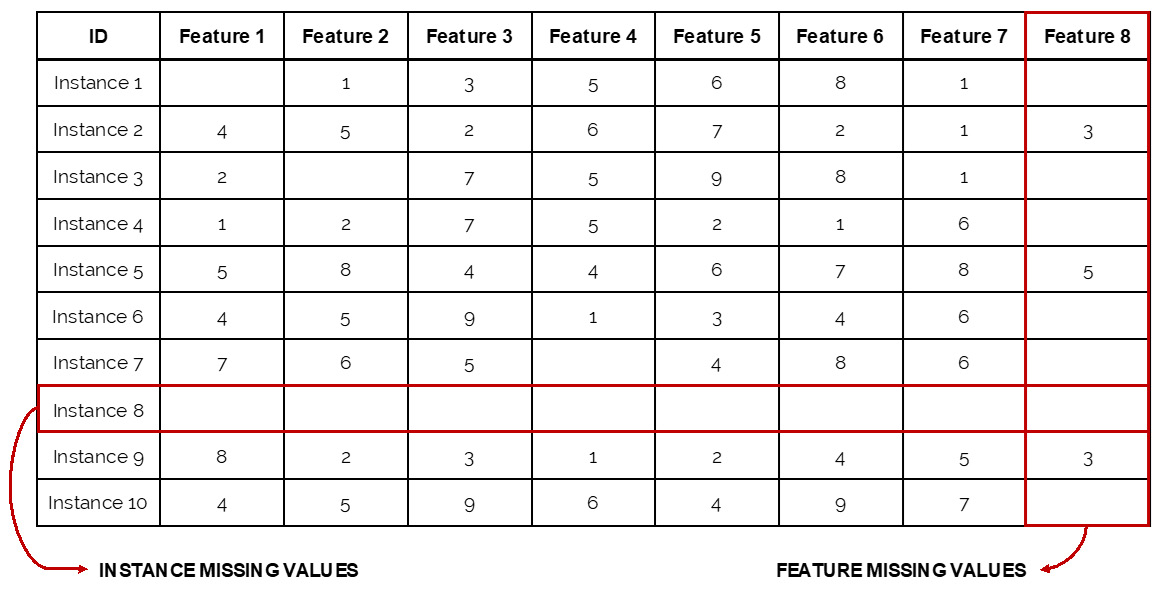Figure 1.6: Example of missing values

The preceding image displays an instance (Instance 8) with no values for any of the features, which makes it useless, and a feature (Feature 8) with seven missing values out of the 10 instances, which means that the feature cannot be used to find patterns among the instances, considering that most of them don't have a value for the feature.

Conventionally, a feature missing more than 5 to 10% of its values is considered to be missing data (also known as a feature with high absence rate), and so it needs to be dealt with. On the other hand, all instances that have missing values for all features should be eliminated as they do not provide any information to the model and, on the contrary, may end up introducing bias.

When dealing with a feature with a high absence rate, it is recommended to either eliminate it or fill it with values. The most popular ways to replace the missing values are as follows:

• Mean imputation: Replacing missing values with the mean or median of the features' available values
• Regression imputation: Replacing missing values with the predicted values that have been obtained from a regression function

Note

A regression function refers to the statistical model that's used to estimate a relationship between a dependent variable and one or more independent variables. A regression function can be linear, logistic, polynomial, and so on.

While mean imputation is a simpler approach to implement, it may introduce bias as it evens out all the instances. On the other hand, even though the regression approach matches the data to its predicted value, it may end up overfitting the model (that is, creating models that learn the training data too well and are not fit to deal with new unseen data) as all the values that are introduced follow a function.

Lastly, when the missing values are found in a text feature such as gender, the best course of action would be to either eliminate them or replace them with a class labeled as uncategorized or something similar. This is mainly because it is not possible to apply either mean or regression imputation to text.

Labeling missing values with a new category (uncategorized) is mostly done when eliminating them would remove an important part of the dataset, and hence would not be an appropriate course of action. In this case, even though the new label may have an effect on the model, depending on the rationale that's used to label the missing values, leaving them empty would be an even worse alternative as it would cause the model to make assumptions on its own.

Note

### Outliers

Outliers are values that are far from the mean. This means that if the values from a feature follow a Gaussian distribution, the outliers are located at the tails.

Note

A Gaussian distribution (also known as a normal distribution) has a bell-shaped curve, given that there is an equal number of values above and below the mean.

Outliers can be global or local. The former group represents those values that are far from the entire set of values for a feature. For example, when analyzing data from all members of a neighborhood, a global outlier would be a person who is 180 years old (as shown in the following diagram (`A`)). The latter, on the other hand, represents values that are far from a subgroup of values of that feature. For the same example that we saw previously, a local outlier would be a college student who is 70 years old (`B`), which would normally differ from other college students in that neighborhood: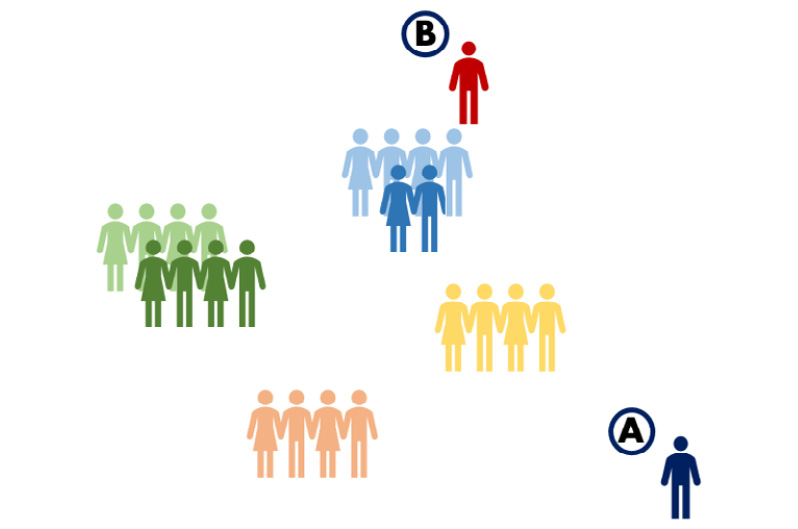Figure 1.7: An image depicting global and local outliers in a dataset

Considering both examples that have been given, outliers do not evaluate whether the value is possible. While a person aged 180 years is not plausible, a 70-year-old college student might be a possibility, yet both are categorized as outliers as they can both affect the performance of the model.

A straightforward approach to detect outliers consists of visualizing the data to determine whether it follows a Gaussian distribution, and if it does, classifying those values that fall between three to six standard deviations away from the mean as outliers. Nevertheless, there is not an exact rule to determine an outlier, and the decision to select the number of standard deviations is subjective and will vary from problem to problem.

For example, if the dataset is reduced by 40% by setting three standard deviations as the parameter to rule out values, it would be appropriate to change the number of standard deviations to four.

On the other hand, when dealing with text features, detecting outliers becomes even trickier as there are no standard deviations to use. In this case, counting the occurrences of each class value would help to determine whether a certain class is indispensable or not. For instance, in clothing sizes, having a size XXS that represents less than 5% of the entire dataset might not be necessary.

Once the outliers have been detected, there are three common ways to handle them:

• Delete the outlier: For outliers that are true values, it is best to completely delete them to avoid skewing the analysis. This may also be a good idea for outliers that are mistakes, that is, if the number of outliers is too large to perform further analysis to assign a new value.
• Define a top: Defining a top may also be useful for true values. For instance, if you realize that all values above a certain threshold behave the same way, you can consider topping that value with a threshold.
• Assign a new value: If the outlier is clearly a mistake, you can assign a new value using one of the techniques that we discussed for missing values (mean or regression imputation).

The decision to use each of the preceding approaches depends on the outlier type and number. Most of the time, if the number of outliers represents a small proportion of the total size of the dataset, there is no point in treating the outlier in any way other than deleting it.

Note

Noisy data corresponds to values that are not correct or possible. This includes numerical (outliers that are mistakes) and nominal values (for example, a person's gender misspelled as "fimale"). Like outliers, noisy data can be treated by deleting the values completely or by assigning them a new value.

## Exercise 1.02: Dealing with Messy Data

In this exercise, we will be using the `tips` dataset from seaborn as an example to demonstrate how to deal with messy data. Follow these steps to complete this exercise:

1. Open a Jupyter Notebook to implement this exercise.
2. Import all the required elements. Next, load the `tips` dataset and store it in a variable called `tips`. Use the following code:
```import seaborn as sns
import numpy as np
import matplotlib.pyplot as plt
3. Next, create a variable called `size` to store the values of that feature from the dataset. As this dataset does not contain any missing data, we will convert the top 16 values of the `size` variable into missing values. Print out the top 20 values of the `age` variable:
```size = tips["size"]
size.loc[:15] = np.nan

Note

A warning may appear at this point, saying A value is trying to be set on a copy of a slice from a DataFrame. This occurs because `size` is a slice of the `tips` dataset, and by making a change in the slice, the dataset is also changed. This is okay as the purpose of this exercise is to modify the dataset by modifying the different features that it contains.

The preceding code snippet creates the size variable as a slice of the dataset, then coverts the top 16 values of the variable into Not a Number (`NaN`), which is the representation of a missing value. Finally, it prints the top 20 values of the variable.

The output will appear as follows: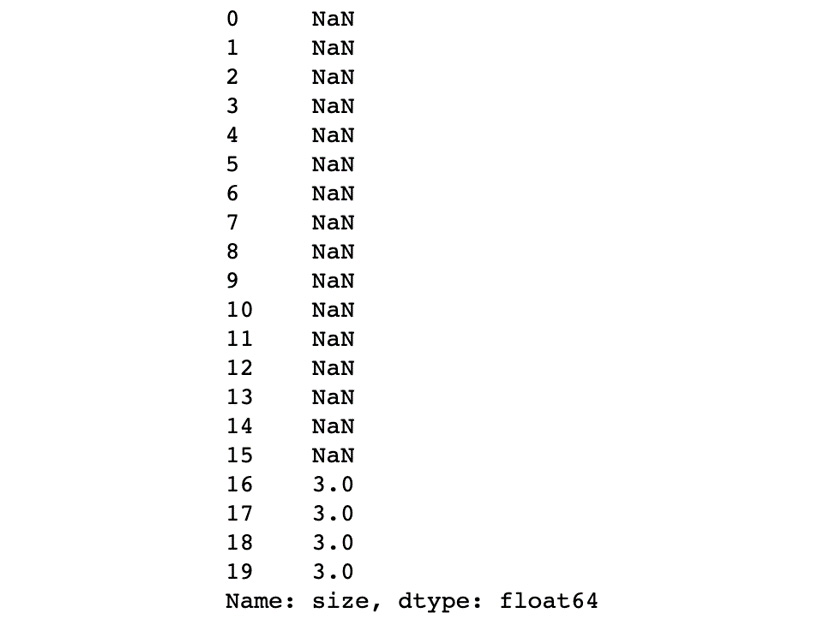Figure 1.8: A screenshot showing the first 20 instances of the age variable

As you can see, the feature contains the `NaN` values that we introduced.

4. Check the shape of the `size` variable:
`size.shape`

The output is as follows:

`(244,)`
5. Now, count the number of `NaN` values to determine how to handle them. Use the `isnull()` function to find the `NaN` values, and use the `sum()` function to sum them all:
`size.isnull().sum()`

The output is as follows:

`16`

The participation of the `NaN` values in the total size of the variable is 6.55%, which can be calculated by dividing the number of missing values by the length of the feature (16/244). Although this is not high enough to consider removing the entire feature, there is a need to handle the missing values.

6. Let's choose the mean imputation methodology to replace the missing values. To do so, compute the mean of the available values, as follows:
```mean = size.mean()
mean = round(mean)
print(mean)```

The mean comes out as `3`.

Note

The mean value (`2.55`) was rounded to its nearest integer since the `size` feature is a measure of the number of persons attending a restaurant.

7. Replace all missing values with the mean. Use the `fillna()` function, which takes every missing value and replaces it with the value that is defined inside the parenthesis. To check that the values have been replaced, print the first 10 values again:
```size.fillna(mean, inplace=True)

Note

When `inplace` is set to `True`, the original DataFrame is modified. Failing to set the parameter to `True` will leave the original dataset unmodified. According to this, by setting `inplace` to `True`, it is possible to replace the `NaN` values for the mean.

The printed output is as follows: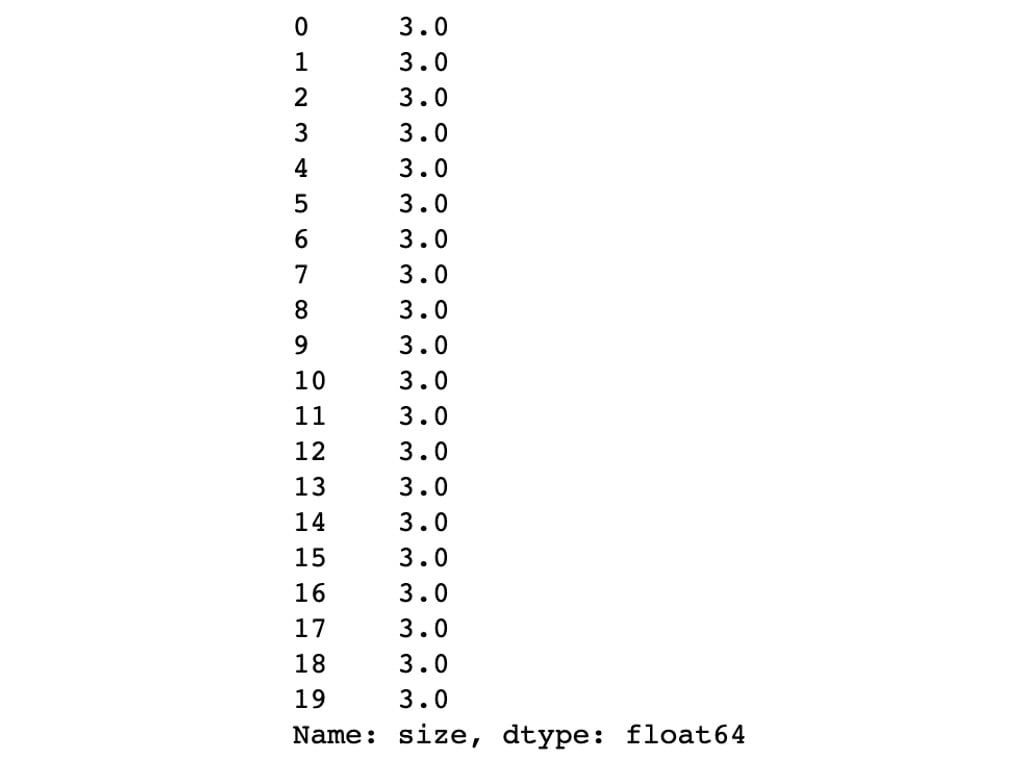Figure 1.9: A screenshot depicting the first 20 instances of the age variable

As shown in the preceding screenshot, the value of the top instances has changed from `NaN` to `3`, which is the mean that was calculated previously.

8. Use Matplotlib to graph a histogram of the `age` variable. Use Matplotlib's `hist()` function, as per the following code:
```plt.hist(size)
plt.show()```

The histogram should look as follows. As we can see, its distribution is Gaussian-like: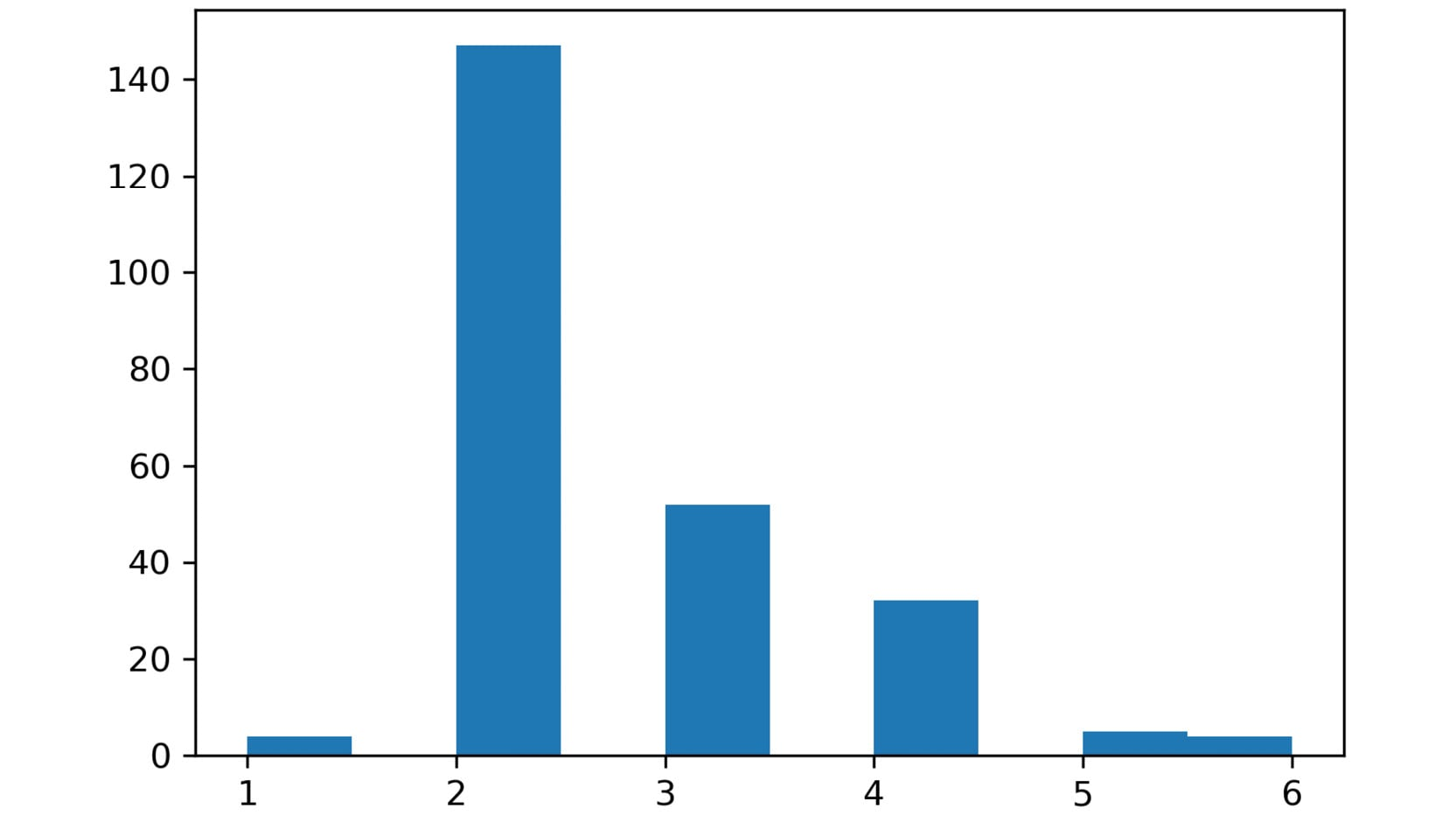Figure 1.10: A screenshot depicting the histogram of the size variable

9. Discover the outliers in the data. Let's use three standard deviations as the measure to calculate the minimum and maximum values.

As we discussed previously, the min value is determined by calculating the mean of all of the values and subtracting three standard deviations from it. Use the following code to set the min value and store it in a variable named `min_val`:

```min_val = size.mean() - (3 * size.std())
print(min_val)```

The min value is around `-0.1974`. According to the min value, there are no outliers at the left tail of the Gaussian distribution. This makes sense, given that the distribution is tilted slightly to the left.

Opposite to the min value, for the max value, the standard deviations are added to the mean to calculate the higher threshold. Calculate the max value, as shown in the following code, and store it in a variable named `max_val`:

```max_val = size.mean() + (3 * size.std())
print(max_val)```

The max value, which comes to around `5.3695`, determines that instances with a size above 5.36 represent outliers. As you can see in the preceding diagram, this also makes sense as those instances are far away from the bell of the Gaussian distribution.

10. Count the number of instances that are above the maximum value to decide how to handle them, as per the instructions given here.

Using indexing, obtain the values in `size` that are above the max threshold and store them in a variable called `outliers`. Then, count the outliers using `count()`:

```outliers = size[size > max_val]
outliers.count()```

The output shows that there are `4` outliers.

11. Print out the outliers and check that the correct values were stored, as follows:
`print(outliers)`

The output is as follows:Figure 1.11: Printing the outliers

As the number of outliers is small, and they correspond to true outliers, they can be deleted.

Note

For this exercise, we will be deleting the instances from the `size` variable to understand the complete procedure of dealing with outliers. However, later, the deletion of outliers will be handled while considering all of the features so that we can delete the entire instance, not just the size values.

12. Redefine the values stored in `size` by using indexing to include only values below the max threshold. Then, print the shape of `size`:
```age = size[size <= max_val]
age.shape```

The output is as follows:

`(240,)`

As you can see, the shape of `size` (calculated in Step 4) has been reduced by four, which was the number of outliers.

Note

To access the source code for this specific section, please refer to https://packt.live/30Egk0o.

You can also run this example online at https://packt.live/3d321ow. You must execute the entire Notebook in order to get the desired result.

You have successfully cleaned a Pandas series. This process serves as a guide for cleaning a dataset later on.

To summarize, we have discussed the importance of preprocessing data, as failing to do so may introduce bias in the model, which affects the training time of the model and its performance. Some of the main forms of messy data are missing values, outliers, and noise.

Missing values, as their name suggests, are those values that are left empty or null. When dealing with many missing values, it is important to handle them by deleting them or by assigning new values. Two ways to assign new values were also discussed: mean imputation and regression imputation.

Outliers are values that fall far from the mean of all the values of a feature. One way to detect outliers is by selecting all the values that fall outside the mean plus/minus three/six standard deviations. Outliers may be mistakes (values that are not possible) or true values, and they should be handled differently. While true outliers may be deleted or topped, mistakes should be replaced with other values when possible.

Finally, noisy data corresponds to values that are, regardless of their proximity to the mean, mistakes or typos in the data. They can be of numeric, ordinal, or nominal types.

Note

Please remember that numeric data is always represented by numbers that can be measured, nominal data refers to text data that does not follow a rank, and ordinal data refers to text data that follows a rank or order.

## Dealing with Categorical Features

Categorical features are features that comprise discrete values typically belonging to a finite set of categories. Categorical data can be nominal or ordinal. Nominal refers to categories that do not follow a specific order, such as music genre or city names, whereas ordinal refers to categories with a sense of order, such as clothing sizes or level of education.

## Feature Engineering

Even though improvements in many ML algorithms have enabled the algorithms to understand categorical data types such as text, the process of transforming them into numeric values facilitates the training process of the model, which results in faster running times and better performance. This is mainly due to the elimination of semantics available in each category, as well as the fact that the conversion into numeric values allows you to scale all of the features of the dataset equally, as will be explained in subsequent sections of this chapter.

How does it work? Feature engineering generates a label encoding that assigns a numeric value to each category; this value will then replace the category in the dataset. For example, a variable called `genre` with the classes `pop`, `rock`, and `country` can be converted as follows: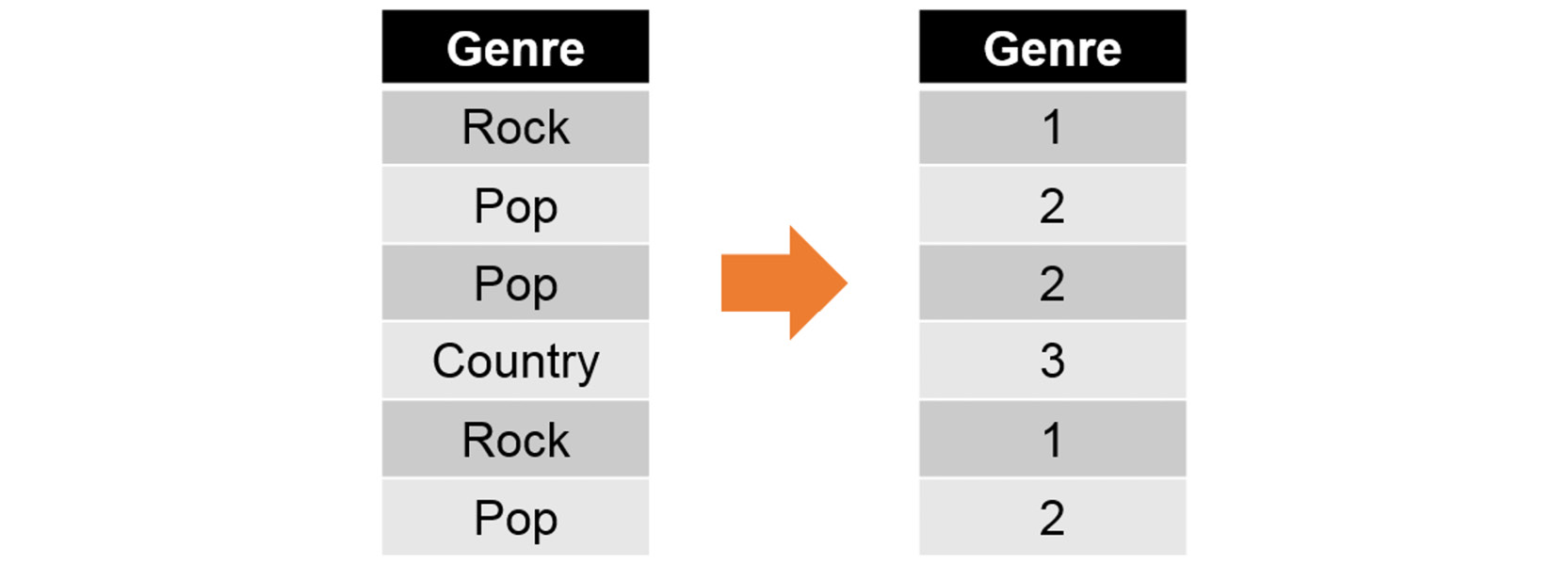Figure 1.12: An image illustrating how feature engineering works

## Exercise 1.03: Applying Feature Engineering to Text Data

In this exercise, we will be converting the text features of the `tips` dataset into numerical data.

Note

Use the same Jupyter Notebook that you created for the previous exercise.

Follow these steps to complete this exercise:

1. Import scikit-learn's `LabelEncoder()` class, as well as the `pandas` library, as follows:
```from sklearn.preprocessing import LabelEncoder
import pandas as pd```
2. Convert each of the text features into numeric values using the class that was imported previously (`LabelEncoder`):
```enc = LabelEncoder()
tips["sex"] = enc.fit_transform(tips['sex'].astype('str'))
tips["smoker"] = enc.fit_transform(tips['smoker'].astype('str'))
tips["day"] = enc.fit_transform(tips['day'].astype('str'))
tips["time"] = enc.fit_transform(tips['time'].astype('str'))```

As per the preceding code snippet, the first step is to instantiate the `LabelEncoder` class by typing in the first line of code. Second, for each of the categorical features, we use the built-in `fit_transform()` method from the class, which will assign a numeric value to each category and output the result.

3. Print out the top values of the `tips` dataset:
`tips.head()`

The output is as follows: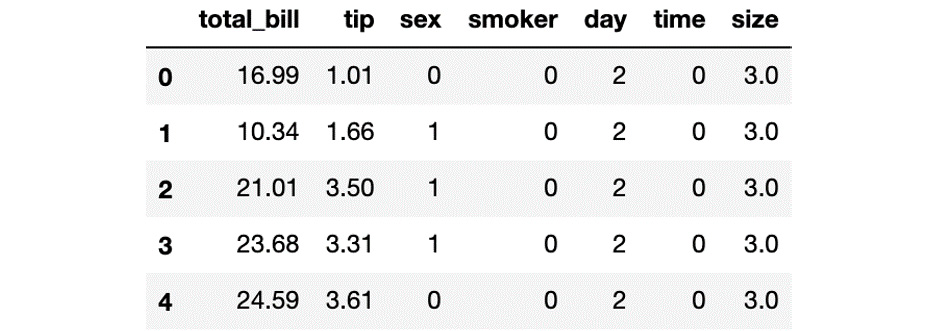Figure 1.13: A screenshot depicting the first five instances of the tips dataset

As you can see, the text categories of the categorical features have been converted into numeric values.

Note

To access the source code for this specific section, please refer to https://packt.live/30GWJgb.

You can also run this example online at https://packt.live/3e2oaVu. You must execute the entire Notebook in order to get the desired result.

You have successfully converted text data into numeric values.

While improvements in ML have made dealing with text features easier for some algorithms, it is best to convert them into numeric values. This is mainly important as it eliminates the complexity of dealing with semantics, not to mention that it gives us the flexibility to change from model to model, without any limitations.

Text data conversion is done via feature engineering, where every text category is assigned a numeric value that replaces it. Furthermore, even though this can be done manually, there are powerful built-in classes and methods that facilitate this process. One example of this is the use of scikit-learn's `LabelEncoder` class.

## Rescaling Data

Rescaling data is important because even though the data may be fed to a model using different scales for each feature, the lack of homogeneity can cause the algorithm to lose its ability to discover patterns from the data due to the assumptions it has to make to understand it, thereby slowing down the training process and negatively affecting the model's performance.

Data rescaling helps the model run faster, without any burden or responsibility to learn from the invariance present in the dataset. Moreover, a model trained over equally scaled data assigns the same weights (level of importance) to all parameters, which allows the algorithm to generalize to all features and not just to those with higher values, irrespective of their meaning.

An example of a dataset with different scales is one that contains different features, one measured in kilograms, another measuring temperature, and another counting the number of children. Even though the values of each attribute are true, the scale of each one of them highly differs from that of the other. For example, while the values in kilograms can go higher than 100, the children count will typically not go higher than 10.

Two of the most popular ways to rescale data are data normalization and data standardization. There is no rule on selecting the methodology to transform data to scale it, as all datasets behave differently. The best practice is to transform the data using two or three rescaling methodologies and test the algorithms in each one of them in order to choose the one that best fits the data based on its performance.

Rescaling methodologies are to be used individually. When testing different rescaling methodologies, the transformation of data should be done independently. Each transformation can be tested over a model, and the best suited one should be chosen for further steps.

Normalization: Data normalization in ML consists of rescaling the values of all features so that they lie in a range between 0 and 1 and have a maximum length of one. This serves the purpose of equating attributes of different scales.

The following equation allows you to normalize the values of a feature:Figure 1.14: The normalization equation

Here, zi corresponds to the ith normalized value and x represents all values.

Standardization: This is a rescaling technique that transforms the data into a Gaussian distribution with a mean equal to 0 and a standard deviation equal to 1.

One simple way of standardizing a feature is shown in the following equation:Figure 1.15: The standardization equation

Here, zi corresponds to the ith standardized value and x represents all values.

## Exercise 1.04: Normalizing and Standardizing Data

This exercise covers the normalization and standardization of data, using the `tips` dataset as an example.

Note

Use the same Jupyter Notebook that you created for the previous exercise.

Follow these steps to complete this exercise:

1. Using the `tips` variable, which contains the entire dataset, normalize the data using the normalization formula and store it in a new variable called `tips_normalized`. Print out the top 10 values:
```tips_normalized = (tips - tips.min())/(tips.max()-tips.min())

The output is as follows: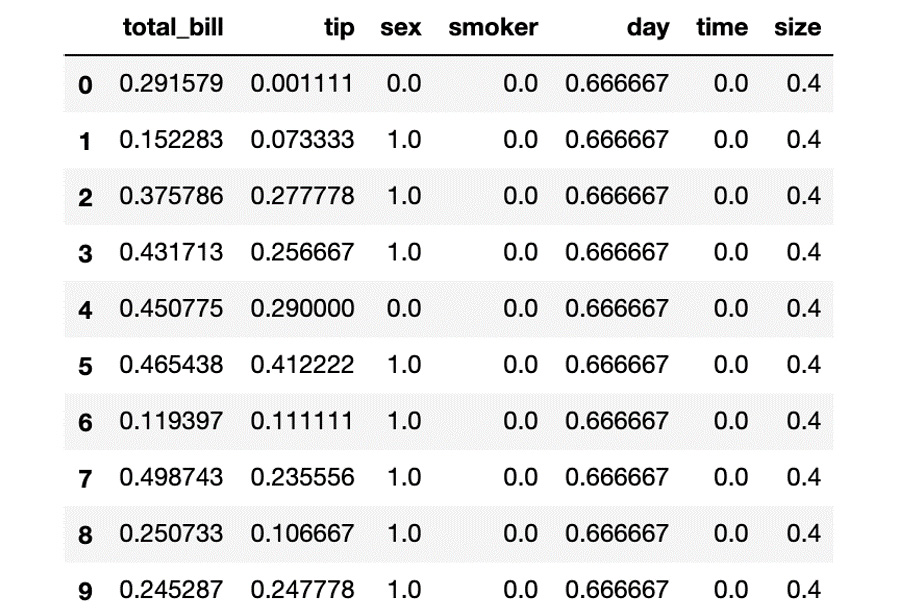Figure 1.16: A screenshot displaying the first 10 instances of the tips_normalized variable

As shown in the preceding screenshot, all of the values have been converted into their equivalents in a range between 0 and 1. By performing normalization for all of the features, the model will be trained on features of the same scale.

2. Again, using the `tips` variable, standardize the data using the formula for standardization and store it in a variable called `tips_standardized`. Print out the top 10 values:
```tips_standardized = (tips - tips.mean())/tips.std()

The output is as follows: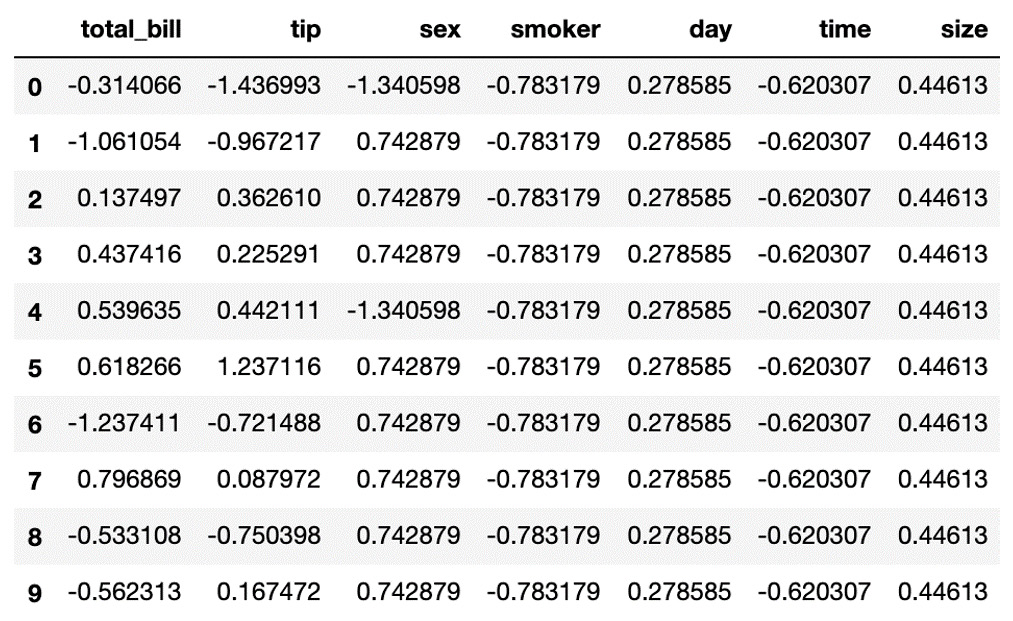Figure 1.17: A screenshot displaying the first 10 instances of the tips_standardized variable

Compared to normalization, in standardization, the values distribute normally around zero.

Note

To access the source code for this specific section, please refer to https://packt.live/30FKsbD.

You can also run this example online at https://packt.live/3e3cW2O. You must execute the entire Notebook in order to get the desired result.

You have successfully applied rescaling methods to your data.

In conclusion, we have covered the final step in data preprocessing, which consists of rescaling data. This process was done in a dataset with features of different scales, with the objective of homogenizing the way data is represented to facilitate the comprehension of the data by the model.

Failing to rescale data will cause the model to train at a slower pace and may negatively affect the performance of the model.

Two methodologies for data rescaling were explained in this topic: normalization and standardization. On one hand, normalization transforms the data to a length of one (from 0 to 1). On the other hand, standardization converts the data into a Gaussian distribution with a mean of 0 and a standard deviation of 1.

Given that there is no rule for selecting the appropriate rescaling methodology, the recommended course of action is to transform the data using two or three rescaling methodologies independently, and then train the model with each transformation to evaluate the methodology that behaves the best.

## Activity 1.02: Pre-processing an Entire Dataset

You are continuing to work for the safety department at a cruise company. As you did great work selecting the ideal target feature to develop the study, the department has decided to commission you for preprocessing the dataset as well. For this purpose, you need to use all the techniques you learned about previously to preprocess the dataset and get it ready for model training. The following steps serve to guide you in that direction:

1. Import `seaborn` and the `LabelEncoder` class from scikit-learn. Next, load the Titanic dataset and create the features matrix, including the following features: `sex`, `age`, `fare`, `class`, `embark_town`, and `alone`.

Note

For this activity, the features matrix has been created using only six features since some of the other features were redundant for this study. For example, there is no need to keep both `sex` and `gender`.

2. Check for missing values and outliers in all the features of the features matrix (`X`). Choose a methodology to handle them.
3. Convert all text features into their numeric representations.
4. Rescale your data, either by normalizing or standardizing it.

Note

The solution for this activity can be found on page 211.

Expected Output: Results may vary, depending on the choices you make. However, you must be left with a dataset with no missing values, outliers, or text features, and with the data rescaled.

# Scikit-Learn API

The objective of the scikit-learn API is to provide an efficient and unified syntax to make ML accessible to non-ML experts, as well as to facilitate and popularize its use among several industries.

## How Does It Work?

Although it has many collaborators, the scikit-learn API was built and has been updated by considering a set of principles that prevent framework code proliferation, where different code performs similar functionalities. On the contrary, it promotes simple conventions and consistency. Due to this, the scikit-learn API is consistent among all models, and once the main functionalities have been learned, it can be used widely.

The scikit-learn API is divided into three complementary interfaces that share a common syntax and logic: the estimator, the predictor, and the transformer. The estimator interface is used for creating models and fitting the data into them; the predictor, as its name suggests, is used to make predictions based on the models that were trained previously; and finally, the transformer is used for converting data.

### Estimator

This is considered to be the core of the entire API, as it is the interface in charge of fitting the models to the input data. It works by instantiating the model to be used and then applies a `fit()` method, which triggers the learning process so that it builds a model based on the data.

The `fit()` method receives the training data as arguments in two separate variables: the features matrix and the target matrix (conventionally called `X_train` and `Y_train`). For unsupervised models, this method only takes in the first argument (`X_train`).

This method creates the model trained to the input data, which can later be used for predicting.

Some models take other arguments besides the training data, which are also called hyperparameters. These hyperparameters are initially set to their default values but can be tuned to improve the performance of the model, which will be discussed in later sections.

The following is an example of a model being trained:

```from sklearn.naive_bayes import GaussianNB
model = GaussianNB()
model.fit(X_train, Y_train)```

First, it is required that you import the type of algorithm to be used from scikit-learn; for example, a Gaussian NaÏve Bayes algorithm (which will be further explored in Chapter 4, Supervised Learning Algorithms: Predicting Annual Income) for classification. It is always good practice to import only the algorithm to be used, and not the entire library, as this will ensure that your code runs faster.

Note

To find the syntax for importing a different model, use the documentation of scikit-learn. Go to the following link, click the algorithm that you wish to implement, and you will find the instructions there: http://scikit-learn.org/stable/user_guide.html.

The second line of code oversees the instantiation of the model and stores it in a variable. Lastly, the model is fitted to the input data.

In addition to this, the estimator also offers other complementary tasks, as follows:

• Feature extraction, which involves transforming input data into numerical features that can be used for ML purposes.
• Feature selection, which selects the features in your data that contribute to the prediction output of the model.
• Dimensionality reduction, which takes high-dimensional data and converts it into a lower dimension.

### Predictor

As explained previously, the predictor takes the model created by the estimator and uses it to perform predictions on unseen data. In general terms, for supervised models, it feeds the model a new set of data, usually called `X_test`, to get a corresponding target or label based on the parameters that were learned while training the model.

Moreover, some unsupervised models can also benefit from the predictor. While this method does not output a specific target value, it can be useful to assign a new instance to a cluster.

Following the preceding example, the implementation of the predictor can be seen as follows:

`Y_pred = model.predict(X_test)`

We apply the `predict()` method to the previously trained model and input the new data as an argument to the method.

In addition to predicting, the predictor can also implement methods that are in charge of quantifying the confidence of the prediction (that is, a numeric value representative of the level of performance of the model). These performance measures vary from model to model, but their main objective is to determine how far the prediction is from reality. This is done by taking an `X_test` with its corresponding `Y_test` and comparing it to the predictions made with the same `X_test`.

### Transformer

As we saw previously, data is usually transformed before being fed to a model. Considering this, the API contains a `transform()` method that allows you to perform some preprocessing techniques.

It can be used both as a starting point to transform the input data of the model (`X_train`), as well as further along to modify data that will be fed to the model for predictions. This latter application is crucial to get accurate results as it ensures that the new data follows the same distribution as the data that was used to train the model.

The following is an example of a transformer that normalizes the values of the training data:

```from sklearn.preprocessing import StandardScaler
scaler = StandardScaler()
scaler.fit(X_train)
X_train = scaler.transform(X_train)```

The `StandardScaler` class standardizes the data that it receives as arguments. As you can see, after importing and instantiating the transformer (that is, `StandardScaler`), it needs to be fit to the data to then effectively transform it:

`X_test = scaler.transform(X_test)`

The advantage of the transformer is that once it has been applied to the training dataset, it stores the values used for transforming the training data; this can be used to transform the test dataset to the same distribution, as seen in the preceding snippet.

In conclusion, we discussed one of the main benefits of using scikit-learn, which is its API. This API follows a consistent structure that makes it easy for non-experts to apply ML algorithms.

To model an algorithm on scikit-learn, the first step is to instantiate the model's class and fit it to the input data using an estimator, which is usually done by calling the `fit()` method of the class. Finally, once the model has been trained, it is possible to predict new values using the predictor by calling the `predict()` method of the class.

Additionally, scikit-learn also has a transformer interface that allows you to transform data as needed. This is useful for performing preprocessing methods over the training data, which can then also be used to transform the testing data to follow the same distribution.

# Supervised and Unsupervised Learning

ML is divided into two main categories: supervised and unsupervised learning.

## Supervised Learning

Supervised learning consists of understanding the relationship between a given set of features and a target value, also known as a label or class. For instance, it can be used for modeling the relationship between a person's demographic information and their ability to pay loans, as shown in the following table: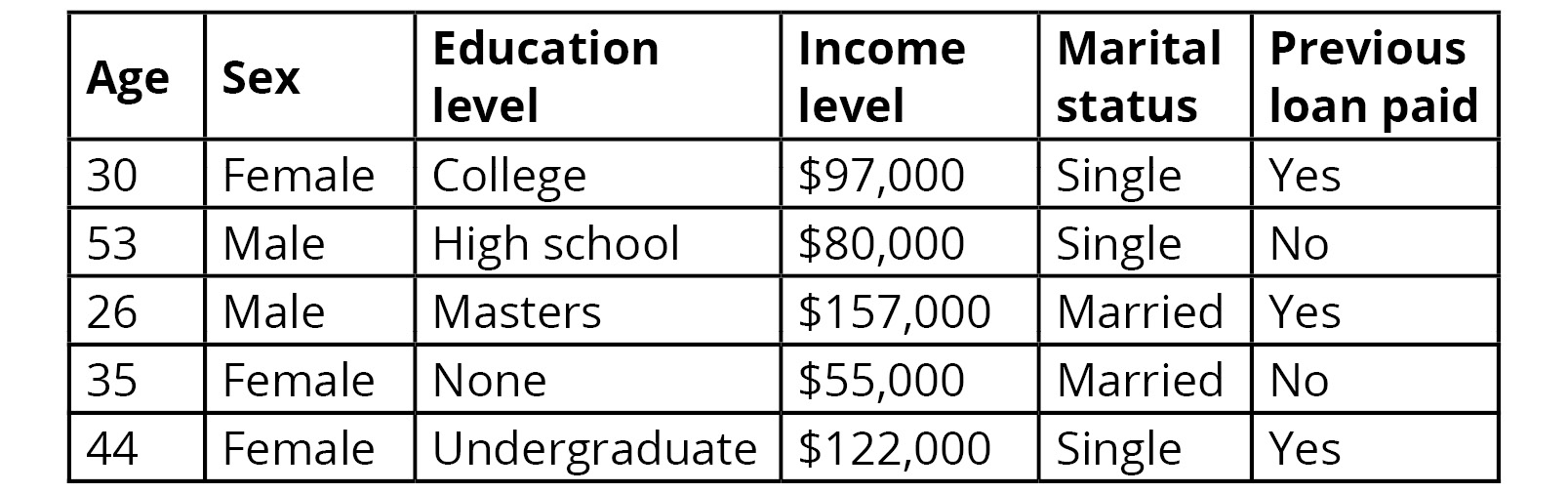Figure 1.18: The relationship between a person's demographic information and the ability to pay loans

Models trained to foresee these relationships can then be applied to predict labels for new data. As we can see from the preceding example, a bank that builds such a model can then input data from loan applicants to determine if they are likely to pay back the loan.

These models can be further divided into classification and regression tasks, which are explained as follows.

Classification tasks are used to build models out of data with discrete categories as labels; for instance, a classification task can be used to predict whether a person will pay a loan. You can have more than two discrete categories, such as predicting the ranking of a horse in a race, but they must be a finite number.

Most classification tasks output the prediction as the probability of an instance to belong to each output label. The assigned label is the one with the highest probability, as can be seen in the following diagram: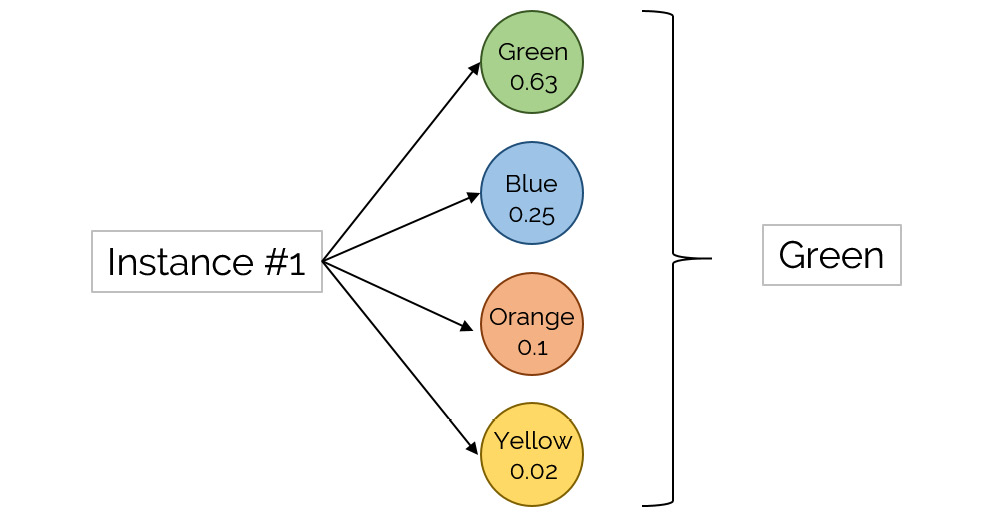Figure 1.19: An illustration of a classification algorithm

Some of the most common classification algorithms are as follows:

• Decision trees: This algorithm follows a tree-like architecture that simulates the decision process following a series of decisions, considering one variable at a time.
• Naïve Bayes classifier: This algorithm relies on a group of probabilistic equations based on Bayes' theorem, which assumes independence among features. It has the ability to consider several attributes.
• Artificial neural networks (ANNs): These replicate the structure and performance of a biological neural network to perform pattern recognition tasks. An ANN consists of interconnected neurons, laid out with a set architecture. They pass information to one another until a result is achieved.

Regression tasks, on the other hand, are used for data with continuous quantities as labels; for example, a regression task can be used for predicting house prices. This means that the value is represented by a quantity and not by a set of possible outputs. Output labels can be of integer or float types:

• The most popular algorithm for regression tasks is linear regression. It consists of only one independent feature (x) whose relationship with its dependent feature (y) is linear. Due to its simplicity, it is often overlooked, even though it performs very well for simple data problems.
• Other, more complex, regression algorithms include regression trees and support vector regression, as well as ANNs once again.

## Unsupervised Learning

Unsupervised learning consists of fitting the model to the data without any relationship with an output label, also known as unlabeled data. This means that algorithms in this category try to understand the data and find patterns in it. For instance, unsupervised learning can be used to understand the profile of people belonging to a neighborhood, as shown in the following diagram: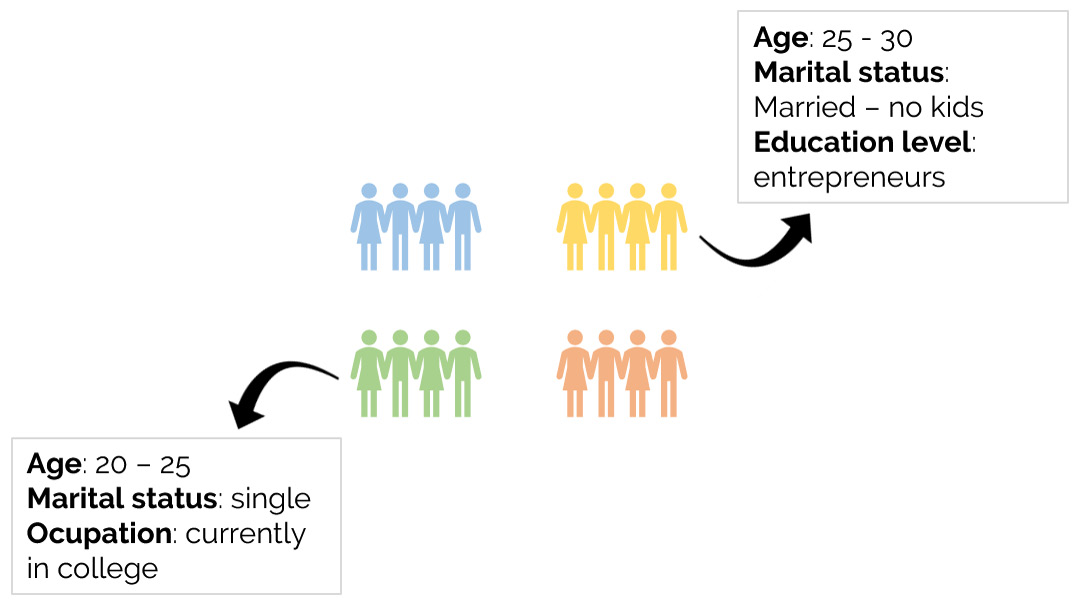Figure 1.20: An illustration of how unsupervised algorithms can be used to understand the profiles of people

When applying a predictor to these algorithms, no target label is given as output. The prediction, which is only available for some models, consists of placing the new instance into one of the subgroups of data that have been created. Unsupervised learning is further divided into different tasks, but the most popular one is clustering, which will be discussed next.

Clustering tasks involve creating groups of data (clusters) while complying with the condition that instances from one group differ visibly from the instances within the other groups. The output of any clustering algorithm is a label, which assigns the instance to the cluster of that label:Figure 1.21: A diagram representing clusters of multiple sizes

The preceding diagram shows a group of clusters, each of a different size, based on the number of instances that belong to each cluster. Considering this, even though clusters do not need to have the same number of instances, it is possible to set the minimum number of instances per cluster to avoid overfitting the data into tiny clusters of very specific data.

Some of the most popular clustering algorithms are as follows:

• k-means: This focuses on separating the instances into n clusters of equal variance by minimizing the sum of the squared distances between two points.
• Mean-shift clustering: This creates clusters by using centroids. Each instance becomes a candidate for centroid to be the mean of the points in that cluster.
• Density-Based Spatial Clustering of Applications with Noise (DBSCAN): This determines clusters as areas with a high density of points, separated by areas with low density.

# Summary

ML consists of constructing models that are able to convert data into knowledge that can be used to make decisions, some of which are based on complicated mathematical concepts to understand data. Scikit-learn is an open source Python library that is meant to facilitate the process of applying these models to data problems, without much complex math knowledge required.

This chapter explained the key steps of preprocessing your input data, from separating the features from the target, to dealing with messy data and rescaling the values of the data. All these steps should be performed before diving into training a model as they help to improve the training times, as well as the performance of the models.

Next, the different components of the scikit-learn API were explained: the estimator, the predictor, and the transformer. Finally, this chapter covered the difference between supervised and unsupervised learning, and the most popular algorithms of each type of learning were introduced.

With all of this in mind, in the next chapter, we will focus on detailing the process of implementing an unsupervised algorithm for a real-life dataset.